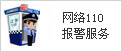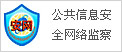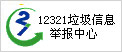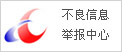﻿ 冷库设计，上海冷库设计
 [免费注册] [会员登录] [会员服务] [加入收藏] 来推广我的公司和服务 | 做VIP会员，获独立网站+营销旺铺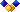我们的服务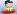会员中心帮助中心 物流导航 冷链商城 物流加盟专题频道 展会频道 冷库频道 制冷频道 农产品频道 水产频道 冷冻频道行业知识 物流学院 网络营销 物流工具 行业动态 市场行情 物流新闻 物流财经 冷链产业 行业新闻 政策法规 特色专题 物 联 网 能源物流 花卉物流 服务产品 会员推广 广告推广 管理助手 招聘求职 物流博客 物流论坛 商务服务 自助建站 物流加盟 冷链商城

 冷链供求冷链产品冷链企业冷藏运输冷库出租冷冻食品冷藏物流制冷设备物流设备热门关键字： 运输 仓储 RFID 冷库 保鲜 冷藏 制冷 冰柜 托盘 叉车 冷藏车 空调 温控..冷库设计建造 >>冷库服务 >> 冷库设计 >>正文

 联系人：电话： EMAIL： QQ/MSN： 商品价格： 区 域： 上海市上海市 发布日期： 2013-10-12 18:34:35 有效天数： 365 冷库设计案例 一、冷库耗冷量的计算 （一） 各间的建筑面积 　由设计说明书可知： 1、 预冷间的建筑面积：180＋96＝276㎡ 2、 冷藏间的建筑面积：192㎡ 3、 冻结间两间的建筑面积：2×48＝96㎡ 4、 贮冰间的建筑面积：192㎡ （二）室内外计算参数 1、窒内计算温度 　冻结间：-23℃ 　冷藏间：-18℃ 　预冷间：-4℃ 　贮冰间：-4℃ 2、窒外计算温度 取夏季空气调节平均温度，查表有湛江夏季空气调节日平均温度为30℃ （三）库房耗冷量的计算 1.计算各房间围护结构传热耗冷量Q1 围护结构传热系数K的确定： 屋顶： 屋盖（上→下 ） （1）40厚预制混凝土板 （2）180厚空气间层 （3）二毡三油 （4）冷底子油一道 （5）20厚水泥沙浆抹面 （6）30厚钢筋混凝土屋盖 （7）1500厚空气间层 （8）聚氯乙烯农用薄膜 （9）250厚聚氯苯乙烯泡沫塑料 （10）二毡三油 （11）冷底子油一道 （12）20厚水泥沙浆抹面 （13）80厚预制钢筋混凝土板 K1= = ≈2.1大卡/米2·时·℃ K2= = ≈2.5大卡/米2·时·℃ K3= = ≈0.14大卡/米2·时·℃ 屋顶总传热系数为：K= = ≈0.125大卡/米2·时·℃ 外墙： （1）20厚水泥沙浆抹面 （2）240厚砖墙 （3）20厚水泥沙浆抹面 （4）冷底子油一道 （5）隔汽层 冻结间：二毡三油 预冷间：一毡二油 储冰间：一毡二油 冷藏间：一毡二油 （6）隔热层 冻结间：250厚聚苯乙烯泡沫塑料 预冷间：100厚聚苯乙烯泡沫塑料 储冰间：150厚聚苯乙烯泡沫塑料 冷藏间：200厚聚苯乙烯泡沫塑料 （7）防潮层 冻结间：二毡三油 预冷间：一毡二油 储冰间：一毡二油 冷藏间：一毡二油 （8）240厚预制混凝土砖墙 （9）20厚水泥沙浆抹面 冻结间：K= ≈0.127大卡/米2·时·℃ 预冷间：K= ≈0.264大卡/米2·时·℃ 贮冰间：K= ≈0.195大卡/米2·时·℃ 冷藏间：K= ≈0.154大卡/米2·时·℃ 内墙： （1）20厚水泥沙浆抹面 （2）240厚预制混凝土砖墙 （3）20厚水泥沙浆抹面 （4）冷底子油一道 （5）一毡二油 （6）隔热层 冻结间：250厚聚苯乙烯泡沫塑料 贮冰间：150厚聚苯乙烯泡沫塑料 冷藏间：200厚聚苯乙烯泡沫塑料 （7）一毡二油 （8）240厚预制混凝土砖墙 （9）20厚水泥沙浆抹面 冻结间：K= ≈0.128大卡/米2·时·℃ 贮冰间：K= ≈0.196大卡/米2·时·℃ 冷藏间：K= ≈0.155大卡/米2·时·℃ 地坪： 地坪（上→下） （1） 80厚钢筋混凝土面层 （2） 15厚水泥沙浆抹面 （3） 一毡二油 （4） 隔热层 冻结间：250厚软木 预冷间：150厚软木 贮冰间：200厚软木 冷藏间：200厚软木 （5）二毡三油 （6）冷底子油一道 （7）15厚水泥沙浆抹面 （8）100厚预制钢筋混凝土板 （9）架空层 （10）60厚100号混凝土垫层 （11）素土夯实 冻结间：K= ≈0.214大卡/米2·时·℃ 预冷间：K= ≈0.319大卡/米2·时·℃ 贮冰间：K= ≈0.253大卡/米2·时·℃ 冷藏间：K= ≈0.253大卡/米2·时·℃ 围护结构传热面积的确定 1． 预冷间的传热面积 　　F北＝36 × 4.2=151.2㎡ F 西＝5×4.2=21㎡　　　　 F西=F东 　　F地=276㎡ F顶=F地 2． 冻结间的传热面积 　　　 F北＝12×4.2＝50.4㎡　　　　　　　F北=F南 　　　　　F 西＝8×4.2＝33.6㎡　　　　　　　 F西=F东 F地=96㎡ F顶=F地 3.冷藏间的传热面积 　　　 F北＝12×4.2＝50.4㎡　　　　　　F北=F南 　　　　　 F东＝16×4.2＝67.2㎡　　　　　　 F西=8×4.2=33.6㎡ F地=192㎡ F顶=F地 4.贮冰间的传热面积 　　　 F南＝12×4.2＝50.4㎡　　　　　　 　　　　　F 西＝16×4.2＝67.2㎡ F地=192㎡ F顶=F地 维护结构耗冷量Q1的计算: 室外计算温度tw=30℃，冻结间的库房温度tn= -23℃，冷藏间的库房温度tn= -18℃ 贮冰间的库房温度tn= -4℃，预冷间的库房温度tn= -4℃　理鱼间的温度tn= 0℃ 温差修正系数：库房与房外大气之间取n=1.0，库房与川堂之间取n=0.7　库房与库房之间取n＝0.7 表有太阳辐射影响的昼夜平均当量温度td有： td北=2.4℃，td南=3.1℃，td东=5.0℃，td西=5.0℃ 各个房间的耗冷量有： 冻结间： 北：Q1=n·K·F·（tZP-tn）=0.127*50.4*[-4-(-23)]*0.7=85大卡/小时 南：Q1=n·K·F·（tZP-tn）=0.127*50.4*[(30+3.1)-(-23)]*1.0= 359大卡/小时 西：Q1=n·K·F·（tZP-tn）=0.127*33.6*[-4-(-23)]*0.7=57大卡/小时 东：Q1=n·K·F·（tZP-tn）=0.127*33.6*[-18-(-23)]*0.7=15大卡/小时 地坪：Q1=n·K·F·（tZP-tn）=0.214*96*[30-(-23)]*1.0=1089大卡/小时 顶：Q1=n·K·F·（tZP-tn）=0.125*96*[30-(-23)]*1.0=636大卡/小时 总Q1=85+359+57+15+1089+636=2241大卡/小时 预冷间： 北：Q1=n·K·F·（tZP-tn）=0.264*151.2*[0-(-23)]*0.7=643大卡/小时 西：Q1=n·K·F·（tZP-tn）=0.264×21×[（30+5）-(-4)] ×1.0=216大卡/小时 东：Q1=n·K·F·（tZP-tn）=0.264×21×[（30+5）-(-4)] ×1.0=216大卡/小时 地坪：Q1=n·K·F·（tZP-tn）=0.319×276×[30-(-4)] ×1.0=2993大卡/小时 屋顶：Q1=n·K·F·（tZP-tn）=0.125×276×[30-(-4)] ×1.0=1173大卡/小时 总Q1=643+216+216+2993+1173=5241大卡/小时 冷藏间： 北：Q1=n·K·F·（tZP-tn）=0.154×50.4×[（-4）-（-18）] ×0.7=76大卡/小时 南：Q1=n·K·F·（tZP-tn）=0.154×50.4×[(30+3.1)-（-18）] ×1.0=397大卡/小时 西：Q1=n·K·F·（tZP-tn）=0.154×33.6×[（-4）-（-18）] ×0.7=51大卡/小时 东：Q1=n·K·F·（tZP-tn）=0.154×67.2×[（30+5）-（-18）] ×1.0=548大卡/小时 地坪：Q1=n·K·F·（tZP-tn）=0.253×192×[30-（-18）] ×1.0=2332大卡/小时 屋顶：Q1=n·K·F·（tZP-tn）=0.125×192×[30-（-18）] ×1.0=1152大卡/小时 总Q1=76+397+51+548+2332+1152=4556大卡/小时 贮冰间： 南：Q1=n·K·F·（tZP-tn）=0.195×50.4×[（30+3.1）-（-4）] ×1.0=365大卡/小时 西：Q1=n·K·F·（tZP-tn）=0.195×67.2×[（30+5）-（-4）] ×1.0=511大卡/小时 屋顶：Q1=n·K·F·（tZP-tn）=0.125×192×[30-（-4）] ×1.0=816大卡/小时 地坪：Q1=n·K·F·（tZP-tn）=0.253×192×[30-（-4）] ×1.0=1652大卡/小时 总Q1=365+511+816+1652=3344大卡/小时 食品冷加工或储存时的耗冷量Q2的计算 食品冷加工或储存时的耗冷量Q2的计算公式为：Q2=Q2a+Q2b+Q2c+Q2d （一） 食品在冷冻加工时的耗冷量： 预冷间： Q2= + = =128大卡/小时 冻结间： Q2= + = =180.8大卡/小时 其中G--食品每日加

【了解更多车源货源信息，请立即扫描中国冷链物流网车源货源二维码，查看车源货源信息或分享给同行好友合作共赢；关注中国冷链物流网官方微信（微信号：cclcn56），还有实用的冷链物流知识、供求信息和优惠活动在等你哦！】车源货源信息 更多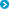冷藏物流专线 更多推荐企业链接